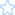Chromium Code Reviews
chromiumcodereview-hr@appspot.gserviceaccount.com (chromiumcodereview-hr) | Please choose your nickname with Settings | Help | Chromium Project | Gerrit Changes | Sign out
(53)

## Side by Side Diff: LayoutTests/fast/forms/number/number-stepup-stepdown-from-renderer-expected.txtIssue 10786036: Merge 122552 - REGRESSION(r119948): [Forms] Spin button Up/Down actions make value to zero for inpu… (Closed) Base URL: http://svn.webkit.org/repository/webkit/branches/chromium/1180/
Patch Set: Created 8 years, 5 months ago
Use n/p to move between diff chunks; N/P to move between comments. Draft comments are only viewable by you.
OLDNEW
1 Check stepping-up and -down for number input from renderer. 1 Check stepping-up and -down for number input from renderer.
2 2
3 On success, you will see a series of "PASS" messages, followed by "TEST COMPLETE ". 3 On success, you will see a series of "PASS" messages, followed by "TEST COMPLETE ".
4 4
5 5
6 Number type 6 Number type
7 Function arguments are (value, step, {min or max}, [stepCount]). 7 Function arguments are (value, step, {min or max}, [stepCount]).
8 Invalid value 8 Invalid value
9 PASS stepUp("", null, null) is "1" 9 PASS stepUp("", null, null) is "1"
10 PASS stepDown("", null, null) is "-1" 10 PASS stepDown("", null, null) is "-1"
(...skipping 31 matching lines...)
42 PASS stepDown("1", "3.40282346e+38", "", 2) is "-3.40282346e+38" 42 PASS stepDown("1", "3.40282346e+38", "", 2) is "-3.40282346e+38"
43 PASS stepUp("-1", "1", "0") is "0" 43 PASS stepUp("-1", "1", "0") is "0"
44 PASS stepUp("0", "1", "0") is "0" 44 PASS stepUp("0", "1", "0") is "0"
45 PASS stepUp("-1", "1", "0", 2) is "0" 45 PASS stepUp("-1", "1", "0", 2) is "0"
46 PASS stepUp("1", "3.40282346e+38", "", 2) is "3.40282346e+38" 46 PASS stepUp("1", "3.40282346e+38", "", 2) is "3.40282346e+38"
47 stepDown()/stepUp() for stepMismatch values 47 stepDown()/stepUp() for stepMismatch values
48 PASS stepUp("1", "2", "") is "2" 48 PASS stepUp("1", "2", "") is "2"
49 PASS input.min = "0"; stepUp("9", "10", "") is "10" 49 PASS input.min = "0"; stepUp("9", "10", "") is "10"
50 PASS stepDown("19", "10", "0") is "10" 50 PASS stepDown("19", "10", "0") is "10"
51 PASS stepUp("89", "10", "99") is "90" 51 PASS stepUp("89", "10", "99") is "90"
52 PASS stepUp("7", "300", "") is "300"
53 PASS stepUp("-7", "300", "") is "0"
54 PASS stepDown("7", "300", "") is "0"
55 PASS stepDown("-7", "300", "") is "-300"
52 Huge value and small step 56 Huge value and small step
53 PASS input.min = ""; stepUp("1e+38", "1", "", 999) is "1e+38" 57 PASS input.min = ""; stepUp("1e+38", "1", "", 999) is "1e+38"
54 PASS input.max = ""; stepDown("1e+38", "1", "", 999) is "1e+38" 58 PASS input.max = ""; stepDown("1e+38", "1", "", 999) is "1e+38"
55 Fractional numbers 59 Fractional numbers
56 PASS input.min = ""; stepUp("0", "0.33333333333333333", "", 3) is "1" 60 PASS input.min = ""; stepUp("0", "0.33333333333333333", "", 3) is "1"
57 PASS stepUp("1", "0.1", "", 10) is "2" 61 PASS stepUp("1", "0.1", "", 10) is "2"
58 PASS input.min = "0"; stepUp("0", "0.003921568627450980", "1", 255) is "1" 62 PASS input.min = "0"; stepUp("0", "0.003921568627450980", "1", 255) is "1"
59 Rounding 63 Rounding
60 PASS stepUp("5.005", "0.005", "", 2) is "5.015" 64 PASS stepUp("5.005", "0.005", "", 2) is "5.015"
61 PASS stepUp("5.005", "0.005", "", 11) is "5.06" 65 PASS stepUp("5.005", "0.005", "", 11) is "5.06"
(...skipping 15 matching lines...) Expand all
77 PASS stepDownExplicitBounds(1, 4, 1, 0) is "0" 81 PASS stepDownExplicitBounds(1, 4, 1, 0) is "0"
78 PASS stepDownExplicitBounds(-4, -1, 1, 0) is "-1" 82 PASS stepDownExplicitBounds(-4, -1, 1, 0) is "-1"
79 PASS stepUpExplicitBounds(-4, -1, 1, 0) is "0" 83 PASS stepUpExplicitBounds(-4, -1, 1, 0) is "0"
80 PASS stepUpExplicitBounds(-100, null, 3, 3) is "5" 84 PASS stepUpExplicitBounds(-100, null, 3, 3) is "5"
81 PASS stepDownExplicitBounds(-100, null, 3, 3) is "2" 85 PASS stepDownExplicitBounds(-100, null, 3, 3) is "2"
82 86
83 PASS successfullyParsed is true 87 PASS successfullyParsed is true
84 88
85 TEST COMPLETE 89 TEST COMPLETE
86 90
OLDNEW
« no previous file with comments | « LayoutTests/fast/forms/number/number-stepup-stepdown-from-renderer.html ('k') | Source/WebCore/platform/Decimal.cpp » ('j') | no next file with comments »

This is Rietveld 408576698